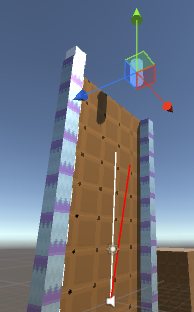# Rigidbody velocity has got different direction than VelocityChange force vector

I want my GameObject to move to direction +y direction with a constant speed.

I chose to apply VelocityChange type fordce to a rigidbody.
The force applied: (0,10,0)
The Rigidbody properties: mass of 1, Use Gravity: false, Is Kinematic: false.

``````public class moveForward : MonoBehaviour
{
public Vector3 force;

void Start ()
{
print("v0: " + GetComponent<Rigidbody>().velocity);

print("f: " + force);
}

int fuCounter = 0

void FixedUpdate()
{
if(0==(fuCounter%50))
{
print("v: " + GetComponent<Rigidbody>().velocity);
}
++fuCounter;
}
}
``````

My expectation was that I measure a velocity which equals the velocity vector I added, namely (0,10,0).
Instead I got (0.0, 9.0, -1.1).

v0: (0.0, 0.0, 0.0)

f: (0.0, 10.0, 0.0)

v: (0.0, 0.0, 0.0)

v: (0.0, 9.0, -1.1)

v: (0.0, 9.0, -1.1)

The force and velocity vector do not point into the same direction:What is the reason of this difference?

I managed to get rid of the problem. I disabled the collider of the plane, which I forgot to do before. Now it works fine.